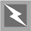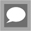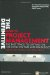# Activity duration estimating

The fourth process group in project time management is activity duration estimating. This is the essence of the entire time management process. The preceding processes have defined the what, when and who for each activity: this is the process that determines how long.## Key Idea

The preceding processes have defined the what, when and who for each activity; this is the process that determines how long.

#### Inputs

There are eight inputs to this process:

• Enterprise environmental factors.

• Organizational process assets.

• Project scope statement.

• Activity lists.

• Activity attributes.

• Activity resource requirements.

• Resource calendar.

• The project management plan.

These inputs have all been described above, as they are used as inputs to the previous process groups. Ideally, the estimating of an activity duration should be carried out by the person doing the work. The role of the project manager is to select the most appropriate estimating tools and techniques and to ensure access to any existing historical or organizational information to assist the estimating process.

#### Tools and techniques

There are five tools and techniques for the project manager to use when estimating the activity durations:

• Expert judgement.

• Analogous estimating.

• Parametric estimating.

• Three-point estimates.

• Reserve analysis.

##### Analogous estimating

Analogous estimating, sometimes called top-down estimating, uses the values of parameters, such as scope, cost, budget and duration from a previous, similar activity as the basis for estimating the same parameter or measure for a future activity. It is frequently used to estimate a parameter when there is a limited amount of detailed information about the project (e.g. estimating in the early phases). Analogous estimating is a form of expert judgement. It is most reliable when the previous activities are similar in fact and not just appearance, and the project team members preparing the estimates have been involved with the previous work.## Analogous estimating

'Analogous Estimating is an estimating technique that uses the values of parameters such as scope, cost, budget and duration or measures of scale such as size, weight and complexity from a previous, similar activity as the basis for estimating the same parameter or measure for a future activity.' PMBOK Guide (p.351)

##### Parametric estimating

Parametric estimating allows the project manager to quantify the activity durations by using a simple (often linear) assumption to relate the amount of work to the productivity rate. The productivity rate can be estimated using historical information or expert judgement. Examples of productivity rates include lines of code per hour, metres of tarmac laid per day, bricks laid per hour, or components machined per week. Johnson famously advised Boswell not to turn his land into an orchard on the basis of a parametric estimate: 'we compute in England a park wall at a thousand pounds per mile ... for a hundred pounds you could have forty-four square yards which is very little'.

##### Three-point estimate

The three-point estimate technique provides an average estimate of the time expected for an activity to be completed. This average is calculated from the most optimistic duration of the activity (O), the most likely (M) and the most pessimistic (P). So, for example, if the optimistic time for a component to be manufactured is 5 days, the pessimistic duration is 12 days and the most likely is 7, the average of these three estimates is 8 days and so 8 days will be used for the duration estimate.

The PERT (Programme Evaluation and Review Technique) estimating technique uses a weighted average to model the duration probability distribution, with M four times more likely to occur than O or P. The PERT distribution is usually normalized by dividing through by 6. For the example given above, the value of the PERT distribution is 7.5 ((5 +(4 x7)+ 12)/6). The mean of the PERT distribution is M. The variance of the PERT distribution (the expected value of the square of the difference between a given value and the mean) is therefore given by ((P O)/6)2 and the standard deviation of the PERT distribution (the absolute square root of the variance) is (P O)/6. So in the above example, the variance is 49/36 and the standard deviation is 7/6.## The PERT distribution

The PERT distribution is given by (P + 4M + O)/6. The variance of the PERT distribution is ((P O)/6)2 and the standard deviation is (P O)/6.

##### Reserve analysis

The final technique, reserve analysis, examines the risks to the project schedule and builds in buffers or time reserves. Building time reserves into a project should not be confused with padding the project. Padding is a term often used to describe extra slack or waiting time that has been built into a project to cover a lack of realistic knowledge during planning.## Reserve analysis

'Reserve Analysis is an analytical technique to determine the essential features and relationships of components in the project management plan to establish a reserve for the schedule duration for a project.' PMBOK Guide (p.372)

#### Outputs

There are two outputs from the activity duration estimating process group:

• Activity duration estimates.

• Updates to the activity attributes.

Top of PageThe Definitive Guide to Project Management: The fast track to getting the job done on time and on budget (2nd Edition)
ISBN: 0273710974
EAN: 2147483647
Year: 2007
Pages: 217
Authors: Sebastian Nokes

Similar book on Amazon